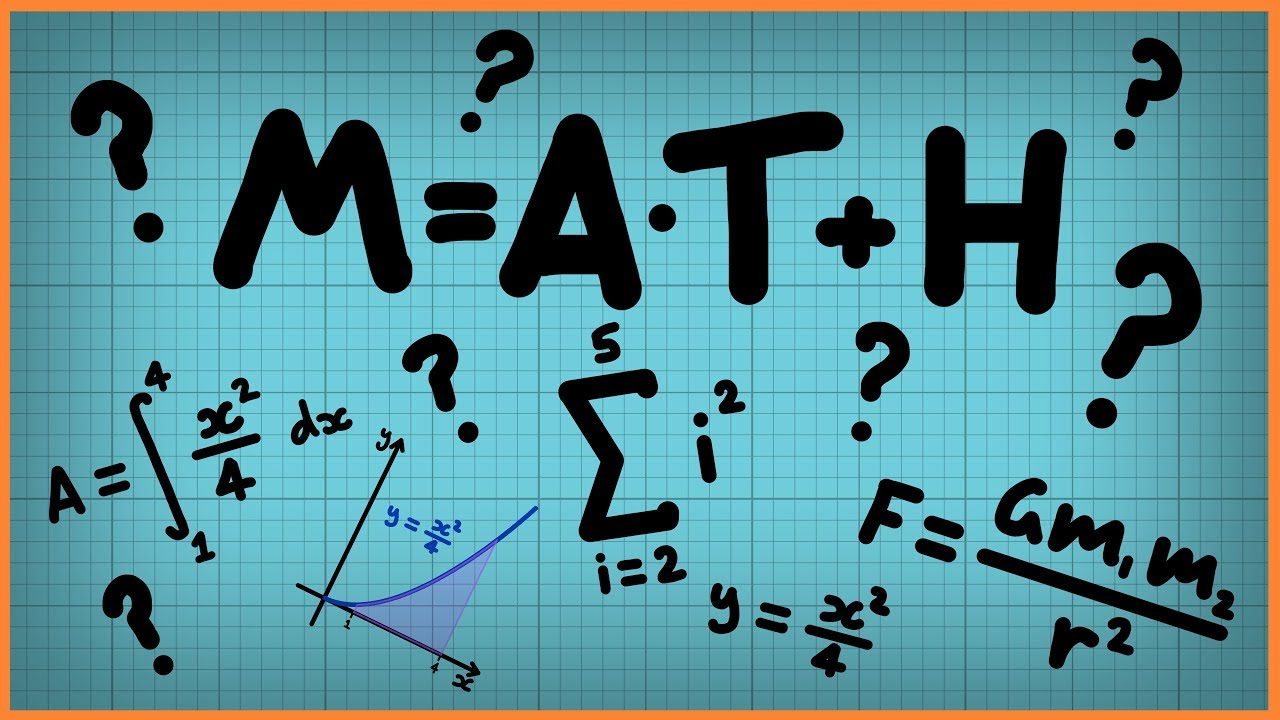Qalaxia Master Bot
0

I found an answer from www.jagranjosh.com

Physics Notes on Units and Measurement for CBSE Class 11

Jun 16, 2017 ... Class 11 Physics notes on Units & Measurement (Chapter 2 of 11th NCERT book ) are available here. ... NCERT Exemplar: Class 11 Physics – Chapter 2 Units and Measurement ... Absolute Error, Relative Error and Percentage Error ... The arithmetic mean of these values is taken as the best possible value ...

For more information, see Physics Notes on Units and Measurement for CBSE Class 11Guru Nanak
0

Given that

Temperature t_1 = 20\degree C \pm 0.5\degree C

Temperature t_2 = 50\degree C \pm 0.5\degree C

Temperature difference \Delta T = t_2 - t_1 = (50\degree C \pm 0.5\degree C) - (20\degree C \pm 0.5\degree C)

The absolute error in the final result is equal to the sum of the absolute errors in the individual quantities when two quantities are added or subtracted.

$\Delta T = [50\degree C - 20\degree C] \pm [0.5\degree C + 0.5\degree C]$

\Delta T = 30\degree C \pm 1\degree C

Hence, Temperature difference \Delta T = 30\degree C \pm 1\degree C